# NCERT Solutions for Chapter 12 Ratio and Proportion Class 6 Maths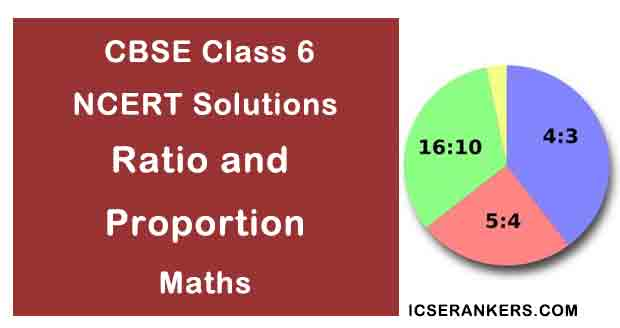### Ratio and Proportion Questions and Answers

 Chapter Name Ratio and Proportion NCERT Solutions Class CBSE Class 6 Textbook Name Maths Related Readings NCERT Solutions for Class 6NCERT Solutions for Class 6 Maths Summary of Ratio and Proportion

Exercise 12.1

Question 1: There are 20 girls and 15 boys in a class.

(a) What is the ratio of number of girls to the number of boys?

(b) What is the ratio of girls to the total number of students in the class?

Solution

(a) The ratio of girls to that of boys = 20/15 = 4/3 = 4:3

(b) The ratio of girls to total students = 20/20 + 15 = 20/35 = 4/7 = 4:7

Question 2: Out of 30 students in a class, like football, 12 like cricket and remaining like tennis. Find the ratio of:

(a) Number of students liking football to number of students liking tennis.

(b) Number of students liking cricket to total number of students.

Solution

Total number of students = 30

Number of students like football = 6

Number of students like cricket = 12

Thus number of students like tennis = 30 – 6 – 12 = 12

(a) The ratio of students like football that of tennis = 6/12 = 1/2 = 1:2

(b) The ratio of students like cricket to that of total students = 12/30 = 2/5 = 2:5

Question 3: See the figure and find the ratio of:

(a) Number of triangles to the number of circles inside the rectangle.

(b) Number of squares to all the figures inside the rectangle.

(c) Number of circles to all the figures inside the rectangle.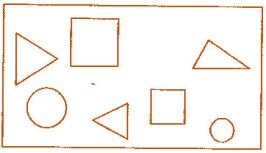Solution

(a) Ratio of number of triangle to that of circles = 3/2 = 3:2

(b) Ratio of number of squares to all figures = 2/7 = 2:7

(c) Ratio of number of circles to all figures = 2/7 = 2:7

Question 4: Distances travelled by Hamid and Akhtar in an hour are 9 km and 12 km. Find the ratio of speed of Hamid to the speed of Akhtar.

Solution

We know that, Speed = Distance/Time

Speed of Hamid = 9 m/1 h = 9 km/h and Speed of Akhtar = 12 m/1 h = 12 km/h

Ratio of speed of Hamid to that of speed of Akhtar = 9/12 = 3/4 = 3:4

Question 5: Fill in the following blanks:

15/18 = /6 = 10/ =  /30

[Are these equivalent ratios?]

Solution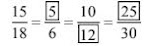Yes, these are equivalent ratios.

Question 6: Find the ratio of the following:

(a) 81 to 108

(b) 98 to 63

(c) 33 km to 121 km

(d) 30 minutes to 45 minutes

Solution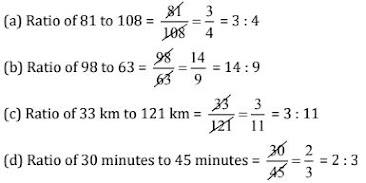Question 7: Find the ratio of the following:

(a) 30 minutes to 1 hour

(b) 40 cm to 1.5 m

(c) 55 paise to ₹ 1

(d) 500 ml to 2 litres

Solution

(a) 30 minutes to 1.5 hour

1.5 hours = 1.5 × 60 = 90 minutes [ 1 hour = 60 minutes]

Now, ratio of 30 minutes to 1.5 hour = 30 minutes : 1.5 hour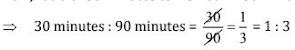(b) 40 cm to 1.5 m

1.5 m = 1.5 × 100 cm = 150 cm [ 1 m = 100 cm]

Now, ratio of 40 cm to 1.5 m = 40 cm : 1.5 m(c) 55 paise to Re. 1

₹ 1 = 100 paise

Now, ratio of 55 paise to ₹1 = 55 paise : 100 paise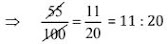(d) 500 ml to 2 litters

2 litres = 2 × 1000 ml = 2000 ml [ 1 litre = 1000 ml]

Now, ratio of 500 ml to 2 litres = 500 ml : 2 litres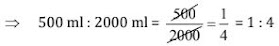Question 8: In a year, Seema earns ₹1,50,000 and saves ₹50,000. Find the ratio of:

(a) Money that Seema earns to the money she saves.

(b) Money that she saves to the money she spends.

Total earning = ₹1,50,000 and Saving = ₹50,000

Solution

Money spent = ₹1,50,000 - ₹50,000 = ₹1,00,000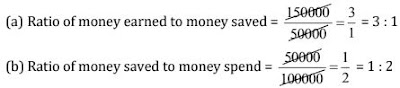Question 9: There are 102 teachers in a school of 3300 students. Find the ratio of the number of teachers to the number of students.

Solution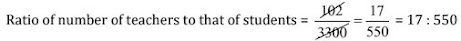Question 10: In a college out of 4320 students, 2300 are girls. Find the ratio of:

(a) Number of girls to the total number of students.

(b) Number of boys to the number of girls.

(c) Number of boys to the total number of students.

solution

Total number of students in school = 4320

Number of girls = 2300

Therefore, number of boys = 4320 – 2300 = 2020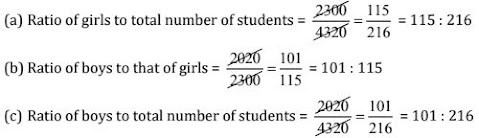Question 11: Out of 1800 students in a school, 750 opted basketball, 800 opted cricket and remaining opted table tennis. If a student can opt only one game, find the ratio of:

(a) Number of students who opted basketball to the number of students who opted table tennis.

(b) Number of students who opted cricket to the number of students opting basketball.

(c) Number of students who opted basketball to the total number of students.

Solution

Total number of students = 1800

Number of students opted basketball = 750

Number of students opted cricket = 800

Therefore, number of students opted tennis = 1800 – (750 + 800) = 250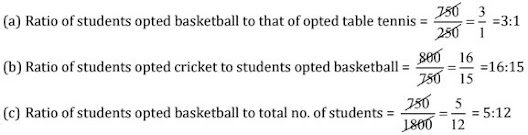Question 12: Cost of a dozen pens is ₹180 and cost of 8 ball pens is ₹56. Find the ratio of the cost of a pen to the cost of a ball pen.

Solution

Cost of a dozen pens (12 pens) = ₹180

Cost of 1 pen = 180/12 = ₹15

Cost of 8 ball pens = ₹56

Cost of 1 ball pen = 56/8 = ₹7

Ratio of cost of one pen to that of one ball pen = 15/7 = 15:7

Question 13: Consider the statement: Ratio of breadth and length of a ball is 2 : 5. Complete the following table that shows some possible breadths and lengths of the hall.

 Breadth of the hall (in meters) 10 __ 40 Length of the hall (in meters) 25 50 __

Solution

Ratio of breadth to length = 2 : 5 = 2/5

Other equivalent ratios are = 2/5 × 10/10 = 20/50 × 2/5 × 20/20 = 40/100

Thus,

 Breadth of the hall (in meters) 10 20 40 Length of the hall (in meters) 25 50 100

Question 14: Divide 20 pens between Sheela and Sangeeta in the ratio 3 : 2.

Solution

Ratio between Sheela and Sangeeta = 3 : 2

Total these terms = 3 + 2 = 5

Therefore, the part of Sheela = 3/5 of the total pens

and the part of Sangeeta = 2/5 of total pens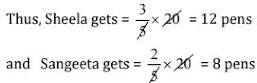Question 15: Mother wants to divide ₹36 between her daughters Shreya and Bhoomika in the ratio of their ages. If the age of Shreya is 15 years and age of Bhoomika is 12 years, find how much Shreya and Bhoomika will get.

Solution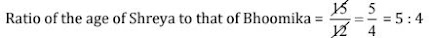Thus, ₹36 divide between Shreya and Bhoomika in the ratio of 5 : 4.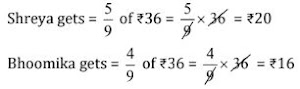Question 16: Present age of father is 42 years and that of his son is 14 years. Find the ratio of:

(a) Present age of father to the present age of son.

(b) Age of the father to the age of the son, when son was 12 years old.

(c) Age of father after 10 years to the age of son after 10 years.

(d) Age of father to the age of son when father was 30 years old.

Solution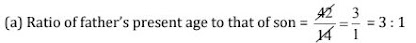(b) When son was 12 years, i.e., 2 years ago, then father was (42 – 2) = 40 years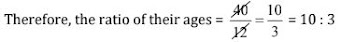(c) Age of father after 10 years = 42 + 10 = 52 years
Age of son after 10 years = 14 + 10 = 24 years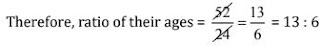(d) When father was 30 years old,
i.e., 12 years ago, then son was (14 – 12) = 2 years old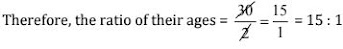Exercise 12.2

Question 1: Determine the following are in proportion:

(a) 15, 45, 40, 120

(b) 33, 121, 9, 96

(c) 24, 28, 36, 48

(d) 32, 48, 70, 210

(e) 4, 6, 8, 12

(f) 33, 44, 75, 100

Solution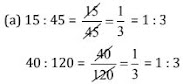Since 15 : 45 = 40 : 120

Therefore, 15, 45, 40, 120 are in proportion.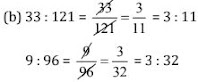Since 33 : 121 ≠ 9 : 96

Therefore, 33, 121, 9, 96 are not in proportion.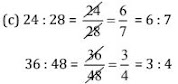Since 24 : 28 ≠ 36 : 48

Therefore, 24, 28, 36, 48 are not in proportion.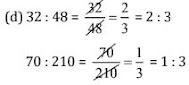Since 32 : 48 ≠ 70 : 210

Therefore, 32, 48, 70, 210 are not in proportion.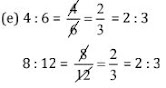Since 4 : 6 = 8 : 12

Therefore, 4, 6, 8, 12 are in proportion.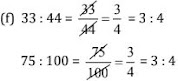Since 33 : 44 = 75 : 100

Therefore, 33, 44, 75, 100 are in ratio.

Question 2: Write True (T) or False (F) against each of the following statements:

(a) 16 : 24 : : 20 : 30

(b) 21 : 6 : : 35 : 10

(c) 12 : 18 : : 28 : 12

(d) 8 : 9 : : 24 : 27

(e) 5.2 : 3.9 : : 3 : 4

(f) 0.9 : 0.36 : : 10 : 4

Solution

(a) 16 : 25 : : 20 : 30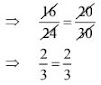Hence, it is True.

(b) 21 : 6 : : 35 : 10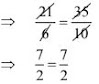Hence, it is True.

(c) 12 : 18 : : 28 : 12Hence, it is False.

(d) 8 : 9 : : 24 : 27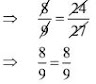Hence, it is True.

(e) 5.2 : 3.9 : : 3 : 4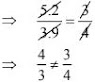Hence, it is False.

(f) 0.9 : 0.36 : : 10 : 4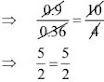Hence, it is True.

Question 3: Are the following statements true:

(a) 40 persons : 200 persons = ₹15 : ₹75

(b) 7.5 litres : 15 litres = 5 kg : 10 kg

(c) 99 kg : 45 kg = ₹44 : ₹20

(d) 32 m : 64 m = 6 sec. : 12 sec.

(e) 45 km : 60 km = 12 hours : 15 hours

Solution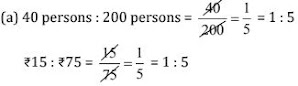Since, 40 persons : 200 persons = ₹15 : ₹75

Hence, the statement is true.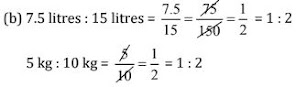Since, 7.5 litres : 15 litres = 5 kg : 10 kg

Hence, the statement is true.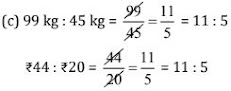Since, 99 kg : 45 kg = ₹44 : ₹20

Hence, the statement is true.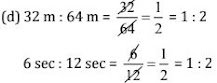Since, 32 m : 64 m = 6 sec : 12 sec

Hence, the statement is true.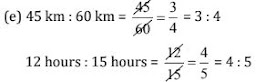Since, 45 km : 60 km ≠ 12 hours : 15 hours

Hence, the statement is not true.

Question 4: Determine if the following ratios form a proportion. Also, write the middle terms and extreme terms where the ratios form a proportion:

(a) 25 cm : 1 m and ₹40 : ₹160

(b) 39 litres : 65 litres and 6 bottles : 10 bottles

(c) 2 kg : 80 kg and 25 g : 625 g

(d) 200 ml : 2.5 ml and ₹4 : ₹50

Solution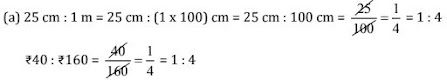Since the ratios are equal, therefore these are in proportion.

Middle terms = 1 m, ₹40 and Extreme terms = 25 cm, ₹160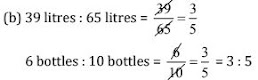Since the ratios are equal, therefore these are in proportion.

Middle terms = 65 litres, 6 bottles and Extreme terms = 39 litres, 10 bottles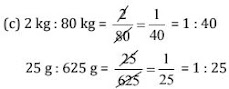Since the ratios are not equal, therefore these are not in proportion.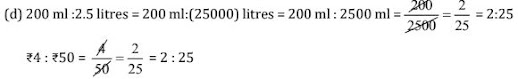Since the ratios are equal, therefore these are in proportion.

Middle terms = 2.5 litres, ₹4 and Extreme terms = 200 ml, ₹50

Exercise 12.3

Question 1: If the cost of 7 m of cloth is ₹294, find the cost of 5 m of cloth.

Solution

Cost of 7 m of cloth = ₹294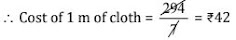∴ Cost of 5 m of cloth = 42 × 5 = ₹210

Thus, the cost of 5 m of cloth is ₹210.

Question 2: Ekta earns ₹1500 in 10 days. How much will she earn in 30 days?

Solution

Earning of 10 days = ₹1500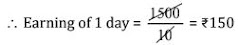∴ Earning of 30 days = 150 × 30 = ₹4500

Thus, the earning of 30 days is ₹4,500.

Question 3: If it has rained 276 mm in the last 3 days, how many cm of rain will fall in one full week (7 days)? Assume that the rain continues to fall at the same rate.

Solution

Rain in 3 days = 276 mm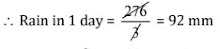∴ Rain in 7 days = 92 × 7 = 644 mm

Thus, the rain in 7 days is 644 mm.

Question 4: Cost of 5 kg of wheat is ₹30.50.

(a) What will be the cost of 8 kg of wheat?

(b) What quantity of wheat can be purchased in ₹61?

Solution

(a) Cost of 5 kg of wheat = ₹30.50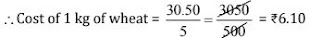∴ Cost of 8 kg of wheat = 6.10 × 8 = ₹48.80

(b) From ₹30.50, quantity of wheat can be purchased = 5 kg

∴ From ₹1, quantity of wheat can be purchased = 5/30.50Question 5: The temperature dropped 15 degree Celsius in the last 30 days. If the rate of temperature drop remains the same, how many degrees will the temperature drop in the next ten days?

Solution

Degree of temperature dropped in last 30 days = 15 degrees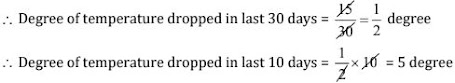Thus, 5 degree Celsius temperature dropped in 10 days.

Question 6: Shaina pays ₹7500 as rent for 3 months. How much does she has to pay for a whole year, if the rent per month remains same?

Solution

Rent paid for 3 months = ₹7500∴ Rent paid for 12 months = 2500 × 12 = ₹30,000

Thus, the total rent of one year is ₹30,000.

Question 7: Cost of 4 dozens bananas is ₹60. How many bananas can be purchased for ₹12.50?

Solution

Cost of 4 dozen bananas = ₹60

Cost of 48 bananas = ₹60 [4 dozen = 4 × 12 = 48]

∵ From ₹60, number of bananas can be purchased = 48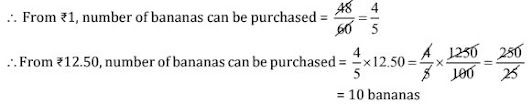Thus, 10 bananas can be purchased for ₹12.50.

Question 8: The weight of 72 books is 9 kg what is the weight of 40 such books?

Solution

The weight of 72 books = 9 kg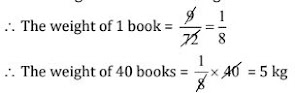Thus, the weight of 40 books is 5 kg.

Question 9: A truck requires 108 litres of diesel for covering a distance of 594 km. How much diesel will be required by the truck to cover a distance of 1650 km?

Solution

For covering 594 km, a truck will be required diesel = 108 litres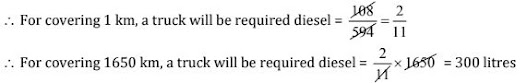Thus, 300 litres diesel required by the truck to cover a distance of 1650 km.

Question 10: Raju purchases 10 pens for ₹150 and Manish buys 7 pens for ₹84. Can you say who got the pen cheaper?

Solution

Raju purchase 10 pens for = ₹150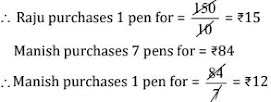Thus, Manish got the pens cheaper.

Question 11: Anish made 42 runs in 6 overs and Anup made 63 runs in 7 overs. Who made more runs per over?

Solution

Anish made in 6 overs = 42 runsThus, Anup made more runs per over.